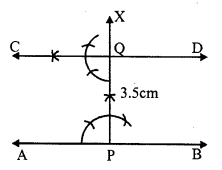## RS Aggarwal Class 7 Solutions Chapter 17 Constructions Ex 17A

These Solutions are part of RS Aggarwal Solutions Class 7. Here we have given RS Aggarwal Solutions Class 7 Chapter 17 Constructions Ex 17A.

Other Exercises

Question 1.
Solution:
Steps of construction :
(i) Draw a line segment AB
(ii) From a point P outside AB, draw a line PQ meeting AB at Q.(iii) At P, draw a line PQ making an angle
∠QPC equal to ∠PQB with the help of compass and ruler and produce it to D.
Then the line CD is parallel to AB. Which is the required line.

Question 2.
Solution:
Steps of construction :
(i) Draw a line AB and take a point P on it.
(ii) From P, draw a perpendicular PX and cut off PQ = 3.5 cm.
(iii) From Q, draw a perpendicular line CD and produce it to both sides.Then, CD is the required line which is parallel to AB.

Question 3.
Solution:
Steps of construction :
(i) Draw a line / and take a point P on it.
(ii) At P, draw a perpendicular line PX and cut off PQ = 4.3 cm.
(iii) From Q, draw a line m which is perpendicular on PX, and produce it to both sides.
Then m is the required line which is parallel to l.

Hope given RS Aggarwal Solutions Class 7 Chapter 17 Constructions Ex 17A are helpful to complete your math homework.

If you have any doubts, please comment below. Learn Insta try to provide online math tutoring for you.